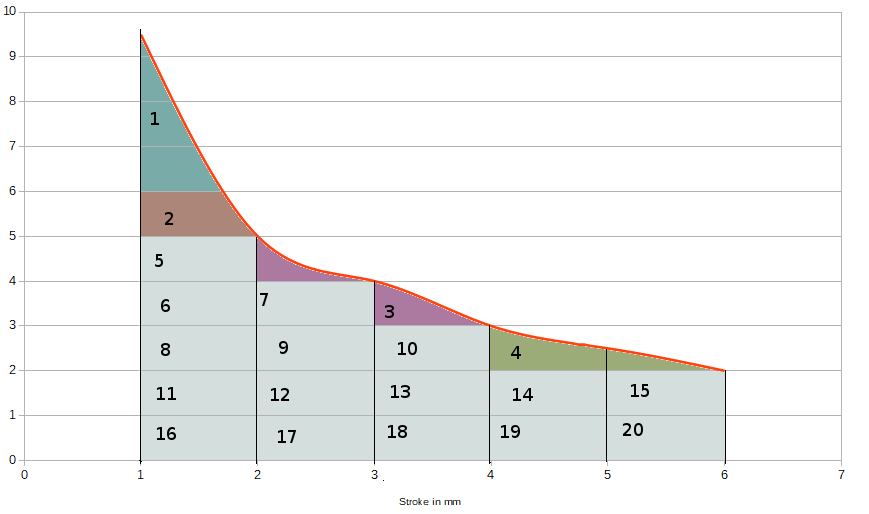# Electricial power output calculation - am I correct?

4 replies to this topic

### #1kevins

kevins
•• Member

• 88 posts
• Joined: August 10

Posted 19 May 2021 - 10:30

Hi, I think this is my first post here, be gentle pleaseI have a question I posted on StackExchange (I forgot about here) and got one answer, but it, the answer is beyond me. Basically, I have a rod that pushes up a travel of 5mm, with a force* between 9.5 and 2kg. It does this in 0.02 seconds. I calculate I can get about 10 watts of electrical power from this - am I in the correct ballpark?

* re force, I know it's measured in Newtons. I simply multiple by 9.8, correct?

I reproduce the question I posted below.

Thanks, Kevin!

I am trying to estimate the electrical power a mechanical machine may generate - is my calculations/understanding correct (to a plus/minus 50% error)?

So, I have a mechanical machine whereby a shaft is pushed up (by what I'd rather not say) and is returned at the top of the stroke by a spring, then the process repeats. Similar to a piston in an ICE.

The 'force' (I'll explain shortly) is not linear along it's travel. It goes from 9.5Kg at 1mm from start, to 2Kg at 6mm. Averaging all my data points I get 4.3Kg over a distance of 5mm.

I understand force is measured in Newtons, so I can just multiple by 9.8?

This process repeats 50 times a second, or every 0.02 seconds.

So, if I connect this to a crankshaft and turn a generator, I believe I will get 10.5 watts of electrical power, assuming no losses, and using my method of simply averaging the force along the travel,

I get 10.5W like so:

Power in watts = work in joules / time

Power in watts = 4.3Kg * 0.005 meters * 9.8 / 0.02 seconds

Edit in response to Mike Jordan's answer.

Unfortunately, I do not understand your answer. This is not my area so am just trying to get a proof of concept before getting the assistance I clearly need.

To try to answer your questions at the end, yes, I'm measuring the force with a load cell calibrated in Kgs.

Since you have the spatial average not the temporal one (right?) - I don't know, I guess the former? Are you saying to multiply the stroke (5mm) by what value? Is that what I did? Is my answer then per stroke?

Advertisement

### #2gruntguru

gruntguru
•• Member

• 7,193 posts
• Joined: January 09

Posted 19 May 2021 - 21:58

10.5 watts is correct. The only uncertainty is how you averaged the force. This needs to be displacement (travel) averaged, not time averaged since work = integral of F x dx not F x dt. The simple way to do this is to plot the force versus travel on graph paper and measure the area under the curve.

10.5 watts is the mechanical power available, a good electrical machine might convert 90% to electricity. the other 10% is lost as heat.

### #3kevins

kevins
•• Member

• 88 posts
• Joined: August 10

Posted 20 May 2021 - 10:54

Hi, many thanks for your help.

I believe I have averaged force (kgs) over distance. I took measurements at 1mm intervals and simply averaged them. Here is a graph.With regard to measuring the area under the curve, do you mean the green area (just noticed, maybe it should extend down to 0 Kg? ) here?In what units would I measure? my x axis is in mm and the y in Kgs - would this not give a wildly different answer?

Sorry for my silly questions, the last time I looked at any maths or physics like this we were starting to see New Romantics on TOTP*

* early 80's if you're not British!

Edited by kevins, 20 May 2021 - 10:55.

### #4gruntguru

gruntguru
•• Member

• 7,193 posts
• Joined: January 09

Posted 23 May 2021 - 22:06

Yes the area under the curve should go down to zero kg.

Your unit of work will be kg.mm. If you want Joules (N.m) you need to multiply by 9.8 and divide by 1,000.

One Watt is one Joule per second. If you divide the work in Joules by the time 9 (in seconds) taken to do that work, you will have power in Watts.

### #5kevins

kevins
•• Member

• 88 posts
• Joined: August 10

Posted 24 May 2021 - 16:18

Thanks again for you help. So I did as you suggested and created this graphic (with the squares actually being rectangles which is just a visual thing):Roughly 20 squares = (20*9.8)/1000 = 0.196 N.m or Joules.

0.196 / 002s = 9.8 Watts

Which is near enough to the original 10.5 W for me at this stage,